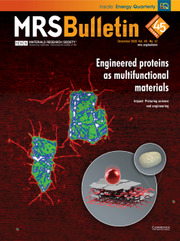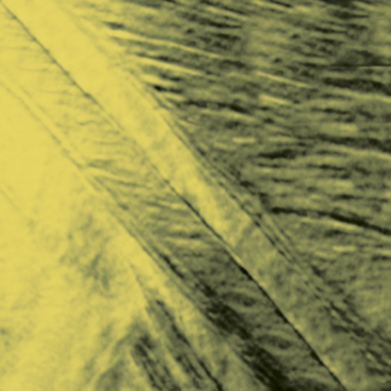Home
Hostname: page-component-568f69f84b-lkk24 Total loading time: 0.261 Render date: 2021-09-22T03:56:04.610Z Has data issue: true Feature Flags: { "shouldUseShareProductTool": true, "shouldUseHypothesis": true, "isUnsiloEnabled": true, "metricsAbstractViews": false, "figures": true, "newCiteModal": false, "newCitedByModal": true, "newEcommerce": true, "newUsageEvents": true }MRS Bulletin

# High piezoelectricity by multiphase coexisting point: Barium titanate derivatives

Published online by Cambridge University Press:  10 August 2018

## AbstractBaTiO3-based lead-free piezoelectric materials have long been known as “a mediocre class of piezoelectric materials.” However, they have seen significant renewed interest in recent years ever since the discovery of high piezoelectricity in Ba(Zr, Ti)O3-(Ba, Ca)TiO3 as well as the related Ba(Sn, Ti)O3-(Ba, Ca)TiO3 and Ba(Hf, Ti)O3-(Ba, Ca)TiO3 systems. The unexpectedly high piezoelectricity in this class of BaTiO3 (BT)-based materials is still not well understood and has stimulated significant research activity. We present a concise discussion of the notions leading to high piezoelectricity in BaTiO3-based systems. In particular, the possible role of a multiphase-coexisting point is highlighted.

## Keywords

Type
Information
MRS Bulletin , August 2018 , pp. 595 - 599

## Access options

Get access to the full version of this content by using one of the access options below. (Log in options will check for institutional or personal access. Content may require purchase if you do not have access.)

## References

Jona, F., Shirane, G., Ferroelectric Crystals (Pergamon Press, Oxford, UK, 1962).Google Scholar
Sluka, T., Tagantsev, A.K., Damjanovic, D., Gureev, M., Setter, N., Nat. Commun. 3, 748 (2012).CrossRefGoogle Scholar
Wada, S., Yako, K., Kakemoto, H., Tsurumi, T., Kiguchi, T., J. Appl. Phys. 98, 014109 (2005).CrossRefGoogle Scholar
Wada, S., Takeda, K., Muraishi, T., Kakemoto, H., Tsurumi, T., Kimura, T., Ferroelectrics 373, 11 (2008).CrossRefGoogle Scholar
Karaki, T., Yan, K., Miyamoto, T., Adachi, M., Jpn. J. Appl. Phys. 46, 97 (2007).CrossRefGoogle Scholar
Shen, Z.Y., Li, J.F., J. Ceram. Soc. Jpn. 118, 940 (2010).CrossRefGoogle Scholar
Ren, X., Nat. Mater. 3, 91 (2004).CrossRefGoogle Scholar
Budimir, M., Damjanovic, D., Setter, N., Phys. Rev. B Condens. Matter 72, 064107 (2005).CrossRefGoogle Scholar
Liu, W., Ren, X., Phys. Rev. Lett. 103, 257602 (2009).CrossRefGoogle Scholar
Xue, D., Zhou, Y., Bao, H., Appl. Phys. Lett. 99, 122901 (2011).CrossRefGoogle Scholar
Zhou, C., Liu, W., Xue, D., Appl. Phys. Lett. 100, 222910 (2012).CrossRefGoogle Scholar
Acosta, M., Novak, N., Jo, W., Acta Mater. 80, 48 (2014).CrossRefGoogle Scholar
Acosta, M., Khakpash, N., Someya, T., Novak, N., Jo, W., Nagata, H., Rossetti, G.A., Rödel, J., Phys. Rev. B Condens. Matter 91, 104108 (2015).CrossRefGoogle Scholar
Brandt, D.R.J., Acosta, M., Koruza, J., Webber, K.G., J. Appl. Phys. 115, 204107 (2014).CrossRefGoogle Scholar
Benabdallah, F., Simon, A., Khemakhem, H., Elissalde, C., Maglione, M., J. Appl. Phys. 109, 124116 (2011).CrossRefGoogle Scholar
Xue, D., Zhou, Y., Bao, H., Gao, J., Zhou, C., Ren, X., J. Appl. Phys. 109, 054110 (2011).CrossRefGoogle Scholar
Xue, D., Gao, J., Zhou, Y., Ding, X., Sun, J., Lookman, T., Ren, X., J. Appl. Phys. 117, 124107 (2015).CrossRefGoogle Scholar
Gao, J., Hu, X., Zhang, L., Li, F., Zhang, L., Wang, Y., Hao, Y., Zhong, L., Ren, X., Appl. Phys. Lett. 104, 252909 (2014).CrossRefGoogle Scholar
Tutuncu, G., Li, B., Bowman, K., Jones, J.L., J. Appl. Phys. 115, 144104 (2014).CrossRefGoogle Scholar
Wang, W., Wang, L.D., Li, W.L., Xu, D., Hou, Y.F., Cao, W.P., Feng, Y., Fei, W.D., Ceram. Int. 40, 14907 (2014).CrossRefGoogle Scholar
Ehmke, M.C., Glaum, J., Hoffman, M., Blendell, J.E., Bowman, K.J., J. Am. Ceram. Soc. 96, 9 (2013).Google Scholar
Acosta, M., Novak, N., Rossetti, G.A., Rödel, J., Appl. Phys. Lett. 107, 142906 (2015).CrossRefGoogle Scholar
Ehmke, M.C., Khansur, N.H., Daniels, J.E., Blendell, J.E., Bowman, K.J., Acta Mater. 66, 340 (2014).CrossRefGoogle Scholar
Li, F., Jin, L., Guo, R., Appl. Phys. Lett. 105, 232903 (2014).CrossRefGoogle Scholar
Acosta, M., Schmitt, L.A., Cazorla, C., Studer, A., Zintler, A., Glaum, J., Kleebe, H.J., Donner, W., Hoffman, M., Rödel, J., Hinterstein, M., Sci. Rep. 6, 28742 (2016).CrossRefGoogle Scholar
Rojas, V., Koruza, J., Patterson, E.A., Acosta, M., Jiang, X., Liu, N., Dietz, C., Rödel, J., J. Am. Ceram. Soc. 100, 4699 (2017).CrossRefGoogle Scholar
Humburg, H.I., Acosta, M., Jo, W., Webber, K.G., Rödel, J., J. Eur. Ceram. Soc. 35, 1209 (2015).CrossRefGoogle Scholar
Zhao, L., Ke, X., Wang, W., Phys. Rev. B Condens. Matter 95, 020101 (2017).CrossRefGoogle Scholar
Acosta, M., Novak, N., Rojas, V., Appl. Phys. Rev. 4, 041305 (2017).CrossRefGoogle Scholar
Ehmke, M.C., Ehrlich, S.N., Blendell, J.E., Bowman, K.J., J. Appl. Phys. 111, 124110 (2012).CrossRefGoogle Scholar
Haugen, A.B., Forrester, J.S., Damjanovic, D., Li, B., Bowman, K.J., Jones, J.L., J. Appl. Phys. 113, 014103 (2013).Google Scholar
Gao, J., Xue, D., Wang, Y., Wang, D., Zhang, L., Wu, H., Guo, S., Bao, H., Zhou, C., Liu, W., Appl. Phys. Lett. 99, 092901 (2011).CrossRefGoogle Scholar
Gao, J., Zhang, L., Xue, D., Kimoto, T., Song, M., Zhong, L., Ren, X., J. Appl. Phys. 115, 054108 (2014).CrossRefGoogle Scholar
Keeble, D.S., Benabdallah, F., Thomas, P.A., Maglione, M., Kreisel, J., Appl. Phys. Lett. 102, 092903 (2013).CrossRefGoogle Scholar
Zhang, L., Zhang, M., Wang, L., Zhou, C., Zhang, Z., Yao, Y., Zhang, L., Xue, D., Lou, X., Ren, X., Appl. Phys. Lett. 105, 162908 (2014).CrossRefGoogle Scholar
Damjanovic, D., Biancoli, A., Batooli, L., Vahabzadeh, A., Trodahl, J., Appl. Phys. Lett. 100, 192907 (2012).CrossRefGoogle Scholar
Zhukov, S., Acosta, M., Genenko, Y.A., Seggern, H.V., J. Appl. Phys. 118, 134104 (2015).CrossRefGoogle Scholar
Noheda, B., Cox, D.E., Shirane, G., Appl. Phys. Lett. 74, 2059 (1999).CrossRefGoogle Scholar
Gao, J.H., Hu, X.H., Wang, Y., Liu, Y.B., Zhang, L.X., Ke, X.Q., Zhong, L.S., Zhao, H., Ren, X.B., Acta Mater. 125, 177 (2017).CrossRefGoogle Scholar
Gao, J., Xue, D., Liu, W., Zhou, C., Ren, X., Actuators 6 (3), 24 (2017).CrossRefGoogle Scholar
Gao, J., Ye, D., Hu, X., Ke, X., Zhong, L., Li, S., Zhang, L., Wang, Y., Wang, D., Wang, Y., Liu, Y., Xiao, H., Ren, X., Europhys. Lett. 115, 37001 (2016).CrossRefGoogle Scholar
Gao, J., Wang, Y., Liu, Y., Hu, X., Ke, X., Zhong, L., He, Y., Ren, X., Sci. Rep. 7, 40916 (2017).CrossRefGoogle Scholar
Gao, J., Liu, Y., Wang, Y., Hu, X., Yan, W., Ke, X., Zhong, L., He, Y., Ren, X., J. Phys. Chem. C 121, 13106 (2017).CrossRefGoogle Scholar
Guo, H., Zhou, C., Ren, X., Tan, X., Phys. Rev. B Condens. Matter 89, 100104 (2014).CrossRefGoogle Scholar
Guo, H., Voas, B.K., Zhang, S., Zhou, C., Ren, X., Beckman, S.P., Tan, X., Phys. Rev. B Condens. Matter 90, 014103 (2014).CrossRefGoogle Scholar
Zakhozheva, M., Schmitt, L.A., Acosta, M., Jo, W., Rödel, J., Kleebe, H.-J., Appl. Phys. Lett. 105, 112904 (2014).CrossRefGoogle Scholar
Zakhozheva, M., Schmitt, L.A., Acosta, M., Guo, H., Jo, W., Schierholz, R., Kleebe, H.J., Tan, X., Phys. Rev. Appl. 3, 064018 (2015).CrossRefGoogle Scholar
Xue, D., Zhou, Y., Gao, J., Ding, X., Ren, X., Europhys. Lett. 100, 17010 (2012).CrossRefGoogle Scholar
Porta, M., Lookman, T., Phys. Rev. B Condens. Matter 83, 174108 (2011).CrossRefGoogle Scholar
Heitmann, A.A., Rossetti, G.A., J. Am. Ceram. Soc. 97, 1661 (2014).CrossRefGoogle Scholar
Yang, T., Ke, X., Wang, Y.. Sci. Rep. 6, 33392 (2016).CrossRefGoogle Scholar
Gao, J., Hao, Y., Ren, S., Kimoto, T., Fang, M., Li, H., Wang, Y., Zhong, L., Li, S., Ren, X., J. Appl. Phys. 117, 084106 (2015).CrossRefGoogle Scholar
Gao, J., Ren, S., Zhang, L., Hao, Y., Fang, M., Zhang, M., Dai, Y., Hu, X., Wang, D., Zhong, L., Li, S., Appl. Phys. Lett. 107, 032902 (2015).CrossRefGoogle Scholar

# Send article to Kindle

Note you can select to send to either the @free.kindle.com or @kindle.com variations. ‘@free.kindle.com’ emails are free but can only be sent to your device when it is connected to wi-fi. ‘@kindle.com’ emails can be delivered even when you are not connected to wi-fi, but note that service fees apply.

Find out more about the Kindle Personal Document Service.

High piezoelectricity by multiphase coexisting point: Barium titanate derivatives
Available formats
×

# Send article to Dropbox

To send this article to your Dropbox account, please select one or more formats and confirm that you agree to abide by our usage policies. If this is the first time you use this feature, you will be asked to authorise Cambridge Core to connect with your <service> account. Find out more about sending content to Dropbox.

High piezoelectricity by multiphase coexisting point: Barium titanate derivatives
Available formats
×

# Send article to Google Drive

To send this article to your Google Drive account, please select one or more formats and confirm that you agree to abide by our usage policies. If this is the first time you use this feature, you will be asked to authorise Cambridge Core to connect with your <service> account. Find out more about sending content to Google Drive.

High piezoelectricity by multiphase coexisting point: Barium titanate derivatives
Available formats
×
×